# Nephroid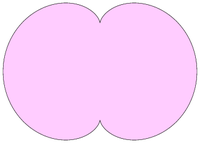Nephroid

The nephroid is a plane curve whose name means kidney-shaped (compare nephrology.) Although the term nephroid was used to describe other curves, it was applied to the curve in this article by Proctor in 1878. This and the information below may be verified in Lockwood, pp. 62–71 (see References).

## Equations for the Nephroid

### Parametric equations

Parametric equations for the nephroid shown above, with cusps on the y-axis, are given by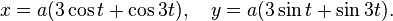$x=a(3\cos t+\cos3t),\quad y=a(3\sin t+\sin3t).$

When the cusps lie on the x-axis, parametric equations are given by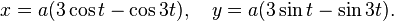$x=a(3\cos t-\cos3t),\quad y=a(3\sin t-\sin3t).$

### Cartesian equation

A Cartesian equation for the nephroid is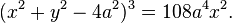$(x^2+y^2-4a^2)^3=108a^4x^2.$

## Properties of the Nephroid

Note: All properties refer to the nephroid as parameterized by the first pair of equations above.

The arc length and area of the nephroid are, respectively,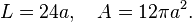$L=24a,\quad A=12\pi a^2.$

The radius of curvature is given by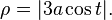$\rho=|3a\cos t|.$

## The Nephroid as...

### ...a caustic of a circle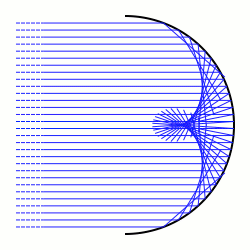Half nephroid as caustic of a semi-circle

A nephroid may be viewed as a caustic of a circle for parallel rays. In other words, if a circle reflected parallel rays of light, the reflected rays would envelope a nephroid. The incoming and reflected rays are shown in blue.

### ...an envelope of circles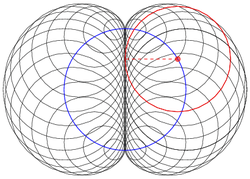Nephroid as envelope of circles

A nephroid may be created as an envelope of circles. Begin with a base circle (blue), then select a point on this circle (red dot). With this point as center, draw a circle (red) tangent to the y-axis. When several such circles are drawn, the image of a nephroid is produced.

Note: In the figure, there are 36 circles whose centers are spaced 10 degrees apart on the base circle.

### ...an envelope of lines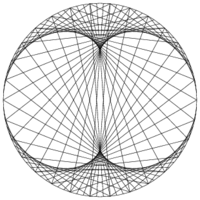Nephroid as envelope of lines

A nephroid may be created as an envelope of lines. Begin with a circle of radius a. For various values of t, join the points which are t degrees and 3t degrees around this circle. In other words, join the points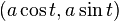$(a\cos t,a\sin t)$ and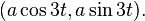$(a\cos 3t,a\sin 3t).$ These lines envelope a nephroid.

Note: In the figure, there are 90 lines corresponding to the parameter t taking on values which are multiples of 4 degrees.

### ...an epicycloid of two cusps

The nephroid is also an epicycloid of two cusps. In the figure, the small blue circle of radius a is externally tangent to the larger black circle of radius 2a. As the smaller circle rolls around the larger circle without slipping, so that the two red arcs are always the same length, the green point traces out a nephroid.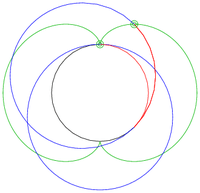Nephroid as epicycloid of two cusps (ii)

This figure shows the nephroid as an epicycloid in another way. The large blue circle of radius 3a is internally tangent to the smaller black circle of radius 2a. As the larger circle rolls around the smaller circle without slipping, so that the two red arcs are always the same length, the green point traces out a nephroid.

## ...of the Nephroid

### The evolute...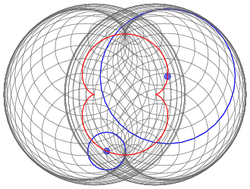Evolute of nephroid

The evolute of the nephroid is another nephroid half as large and rotated 90 degrees. The original nephroid is visible as an envelope of its osculating circles (gray). Two osculating circles are shown (blue). The centers of these circles are seen to lie on the evolute (smaller nephroid, red).

Note: In the figure, there are 60 circles corresponding to the parameter t taking on values which are multiples of 6 degrees.

### The involute...

Because the evolute of the nephroid is another nephroid, so the involute of the nephroid is also another nephroid. The original nephroid (envelope of gray circles) in the previous image is the involute of the smaller red nephroid.

### The inverse...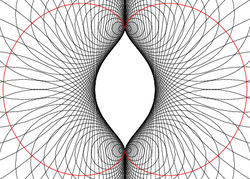Inverse of nephroid

The inverse with respect to the origin of the nephroid (red) is the curve shown as an envelope of circles (black). These circles are inverse to those in the above figure describing the nephroid as an envelope of circles.

Inverting about a circle of radius a, a Cartesian equation for the inverse is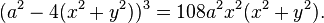$(a^2-4(x^2+y^2))^3=108a^2x^2(x^2+y^2).$

## References

Lockwood, E. H., A Book of Curves, Cambridge University Press, 1961.